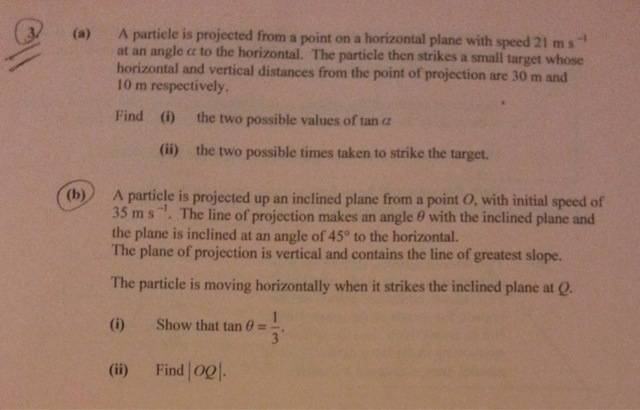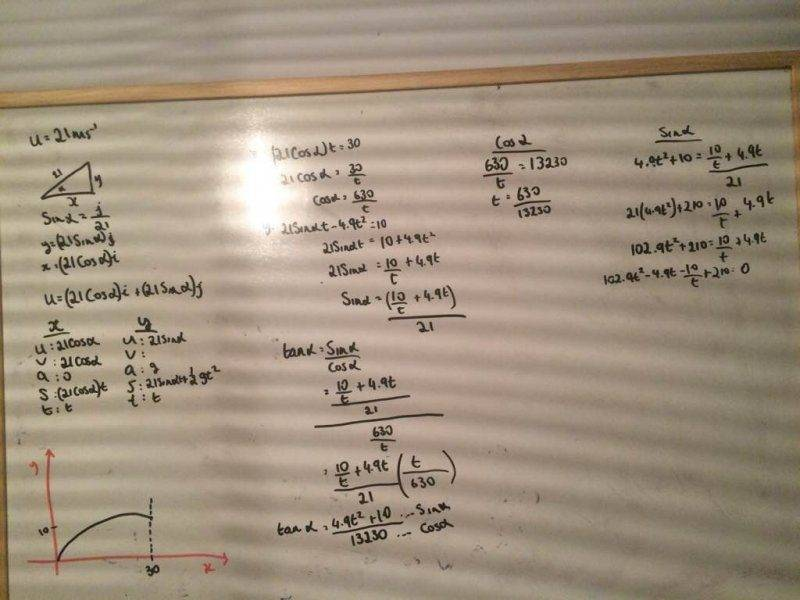# Projectiles problem, help needed

Here is the problem (Q3 (A));Here's what I have so far;Any help would be appreciated, thank you!

RUber
Homework Helper
It appears you made an algebra error when you went from ## 21 \cos \alpha = \frac {30}{ t} ## to ## \cos \alpha = \frac {630}{ t} ##.
Also, I would first find your time of impact based on your horizontal component.
With that time of impact, you can make an expression that is in terms of alpha only.

It appears you made an algebra error when you went from ## 21 \cos \alpha = \frac {30}{ t} ## to ## \cos \alpha = \frac {630}{ t} ##.
Also, I would first find your time of impact based on your horizontal component.
With that time of impact, you can make an expression that is in terms of alpha only.

Yes I realised this after I posted, I'll try that, thanks!

RUber
Homework Helper
I made a substition of x = cos^2(alpha) and manipulated it into a quadratic equation to get the two solutions.
part b comes right out of part a if you have already solved for time of impact.

TSny
Homework Helper
Gold Member
Aaron,

Something else to note: If you have the expression ##\tan \alpha = \frac{A}{B}##, then you cannot conclude that ##\sin \alpha = A## and ##\cos \alpha = B##.

I made a substition of x = cos^2(alpha) and manipulated it into a quadratic equation to get the two solutions.
part b comes right out of part a if you have already solved for time of impact.

Could you elaborate on this a bit? Not quite sure what you mean..

RUber
Homework Helper
Do you have an expression that can be written as some trig functions of alpha = 0?
Use sin = sqrt(1-cos^2) to convert everything in terms of cos.